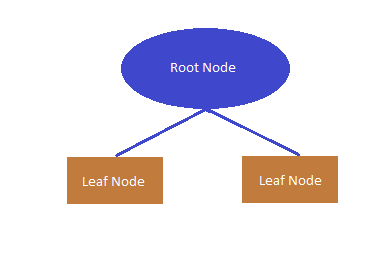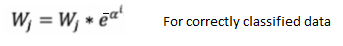top of pageSearch

AdaBoost is adaptive learning algorithm, which learns from calling weak learning algorithms repeatedly in a sequential way. It adapts to the error rates of individual weak hypothesis. This is the basis of its name- "ada" means adaptive. It is an ensembled boosting model.

First let us see what is boosting and then understand how AdaBoost works.

## Boosting

Boosting is a technique of combining set of weak learners into a strong learner.

A weak learner is a classifier whose performance is poor (accuracy is slightly better than a random guess). In contrast, a strong learner is a classifier with arbitrarily high accuracy.

Boosting idea is to train weak learners sequentially, each trying to correct its predecessor. This means, the algorithm is always going to learn something which is not always completely accurate but by sequentially correcting the errors, it improves the prediction power.image towardsdatascience

The way in which the weak learners are selected and combined in boosting depends on the methodology/algorithm. e.g., AdaBoost, Gradientboost, XGboost.

AdaBoost algorithm works by first fitting a weak classifier on original dataset producing an output hypothesis and then iteratively reweighting the misclassified data to fit the next weak classifier. Each weak learner is assigned a coefficient such that the sum of the training error of the resulting boosted classifier is minimized.

Training a AdaBoost classifier consist of iteratively learning weak classifiers that are weighted in a way that is related to the weak learner’s performance and adding them to the final strong classifier. After a weak learner is added, the input data weights are adjusted, known as "re-weighting".

Re-weighting means the input data that is misclassified would gain more weight and the correctly classified data would lose weight. Thus, the next weak learners focus more on the data that previous weak learner misclassified.

The commonly used weak learners in AdaBoost classifier are decision trees with single split called decision stumps.## Coefficient of the classifier

AdaBoost trains a sequence of classifiers(weak learner) with re-weighted sample data, generating coefficients α for individual classifiers based on errors. This coefficient determines how much this weak classifier contributes to the final combined classifier.

α looks at the errors that classifier make. As we can see from the below graph, when error is high, α is smaller i.e., less importance of the classifier with errors in the voting. When error is low, α is large, which means higher importance in the voting.image coderspacket

The prediction of the final combined classifier is done by weighted voting. When the test data is passed through each classifier, the predicted output would be the maximum of the sum of the coefficient of each classifier classifying the data to a particular class.

This process can be understood in detail from the below example.

## Example

Suppose there are 2 classes blue and brown and a sample dataset for training has 8 points (4 black and 4 yellow) and if the output should classify all black points to the blue class and all yellow points to the brown class:

For the first iteration, all the points are having equal weight of 1/8. When data is passed to the 1st weak classifier, it predicts 3 points to the blue class and 5 points to the brown class.Thus the 1st classifier is misclassifying 1 black point into brown class. So, for 2nd iteration, this misclassified black point weight is increased and weights of other points are reduced. i.e., the weight of misclassified black point is increased to 5/12 and the weights of remaining points reduced to 1/12 (at any time, sum of weights of all sample data should always be 1.So, normalization of weights is performed).

Now the new weighted dataset is passed through the 2nd classifier.The 2nd classifier classifies 5 points to the blue class and 3 points to the brown class. Though this classifier correctly classifies the previously misclassified black point to the blue class, but this classifier is misclassifying one yellow point to brown class. So, again the weights are adjusted such that the weight of wrongly classified yellow point in increased and weight of correctly classified data is decreased i.e., the new updated weights would be 1/16,1/16,1/16,6/16,4/16,1/16,1/16,1/16 (weights are normalized to make the sum as 1).

This training process continues until all the training data points are correctly classified into the blue and brown classes.

In the above example after training, if we get 5 weak classifiers finally, each classifier is having α(coefficient) as 0.8, 0.6, 0.3, 0.5, 0.4.

Suppose for a test data point if 2 classifiers with α 0.4 and 0.6 predict that the test data point belong to the blue class and 3 classifiers with α 0.8,0.3,0.5 predicts that the test point belongs to the brown class then the final prediction would be the class with highest weighted vote. i.e., the class which has maximum sum of α.

i.e., maximum of 0.4 +0.6 =1.0 (for blue class) and 0.3+0.5+0.4 = 1.6 (for brown class). So, the final output is that the point belongs to brown class(as the sum=1.6).

## Pseudocode

Here is the pseudocode for AdaBoost algorithm.

1. Initialize weights of input data W: W1, W2, W3, …, Wn=1/n

2. For each iteration, i = 1: T

• Train each weak learning algorithm using data weighted by W. This produces weak classifier hypothesis C.

• Calculate coefficient of classifier α usingWhere Error is Sum of weights of misclassified data

• Update weights as:• Normalize the weights so that sum of the weights is equal to 1

3. Output of the model is done by weighted voting. Prediction is maximum of sum of the coefficient of each classifier classifying the data to a particular class k.## Implementation in Python

Scikit-learn offers implementation of AdaBoost classifier. All we need to do is populate AdaBoost classifier with data and pass parameters like which weak learning algorithm to be used and how many iterations of boosting etc. And everything is done inside the AdaBoost classifier.

class sklearn.ensemble.AdaBoostClassifier(base_estimator, n_estimators, learning_rate, algorithm, random_state)

Example code snippet from kaggle:Below are the parameters of the AdaBoost classifier:

1. base_estimator: This parameter tells AdaBoost about which weak learning classifier to be chosen. Default is Decision tree classifier with max_depth = 1.

2. n_estimators: This parameter tells the maximum number of estimators(iterations) at which boosting is terminated. Default value is 50.

3. learning_rate: This parameter tells how much learning a classifier contributes to the weight of the input data. If learning rate is smaller then input weights are reduced by learning_rate times forcing the model to train slower. Default value is 1. Low learning_rate requires more n_estimators.

4. algorithm: This parameter tells which boosting algorithm SAMME or SAMME.R to be selected. SAMME.R requires the base_estimator to support calculation of class probabilities. SAMME supports multi classification. Default is SAMME.R.

5. random_state: This parameter effects the reproducibility of the results returned. Default is None.

## Pros and Cons of AdaBoost

Pros:

1. It is fast, simple and easy to program.

2. Reduced Bias

3. It is extended to problems beyond binary classification

Cons:

1. It is vulnerable to noise

2. May lead to overfitting of data

### Sources

• Boosting Algorithms Explained:

• Understanding and Implementing AdaBoost Algorithm in Python: